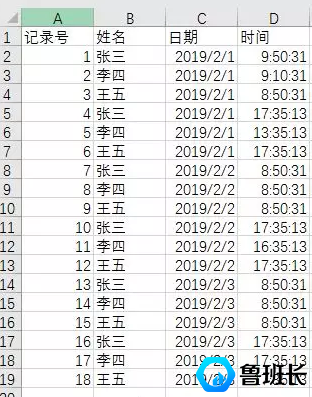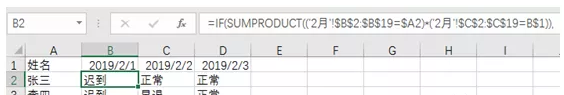## 如何快速整理考勤记录结果！=IF(SUMPRODUCT(('2月'!\$B\$2:\$B\$19=\$A2)*('2月'!\$C\$2:\$C\$19=B\$1)),IF(SUMPRODUCT(('2月'!\$B\$2:\$B\$19=\$A2)*('2月'!\$C\$2:\$C\$19=B\$1)*('2月'!\$D\$2:\$D\$19<9/24)),IF(SUMPRODUCT(('2月'!\$B\$2:\$B\$19=\$A2)*('2月'!\$C\$2:\$C\$19=B\$1)*('2月'!\$D\$2:\$D\$19>17.5/24)),"正常","早退"),"迟到"),"旷工")

=IF(SUMPRODUCT((记录表的姓名区域=\$A2)*(记录表的日期区域=B\$1)),IF(SUMPRODUCT((记录表的姓名区域=\$A2)*(记录表的日期区域=B\$1)*(记录表的时间区域<早上打卡时间)),IF(SUMPRODUCT((记录表的姓名区域=\$A2)*(记录表的日期区域=B\$1)*(记录表的时间区域>晚上打卡时间)),"正常","早退"),"迟到"),"旷工")

### 工地安全教育资讯查看更多》

#### 热门标签